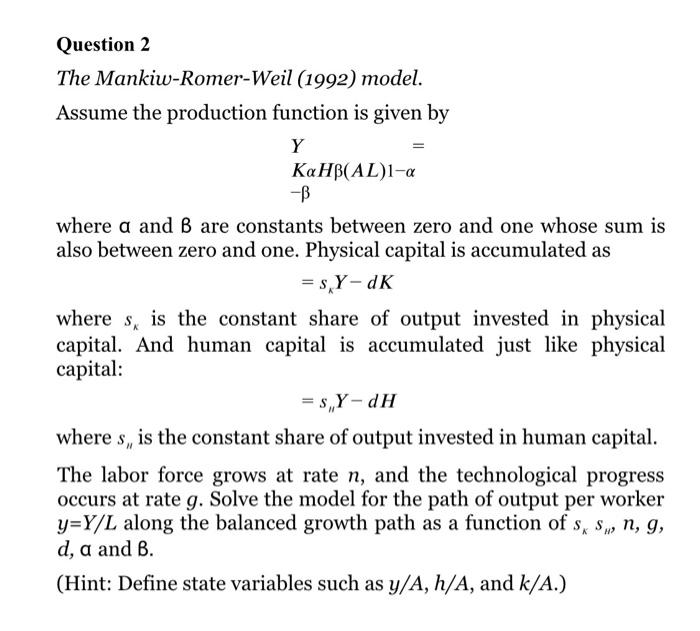### Create an Account

Already have account?

### Forgot Your Password ?

Home / Questions / The Mankiw-Romer-Weil (1992) model. Assume the production function is given by Y KaHB(AL)1...

# The Mankiw-Romer-Weil (1992) model. Assume the production function is given by Y KaHB(AL)1-a -B where a and B are constants between zero and one whose sum is also between zero and one. Physical

The Mankiw-Romer-Weil (1992) model. Assume the production function is given by Y KaHB(AL)1-a -B where a and B are constants between zero and one whose sum is also between zero and one. Physical capital is accumulated as = SY-DK where s, is the constant share of output invested in physical capital. And human capital is accumulated just like physical capital: = s, Y-dH where s, is the constant share of output invested in human capital. The labor force grows at rate n, and the technological progress occurs at rate g. Solve the model for the path of output per worker y=Y/L along the balanced growth path as a function of sx Syn, g, d, a and B. (Hint: Define state variables such as y/A, h/A, and k/A.)Jun 05 2021 View more View Less

#### Answer (Solved)Subscribe To Get Solution